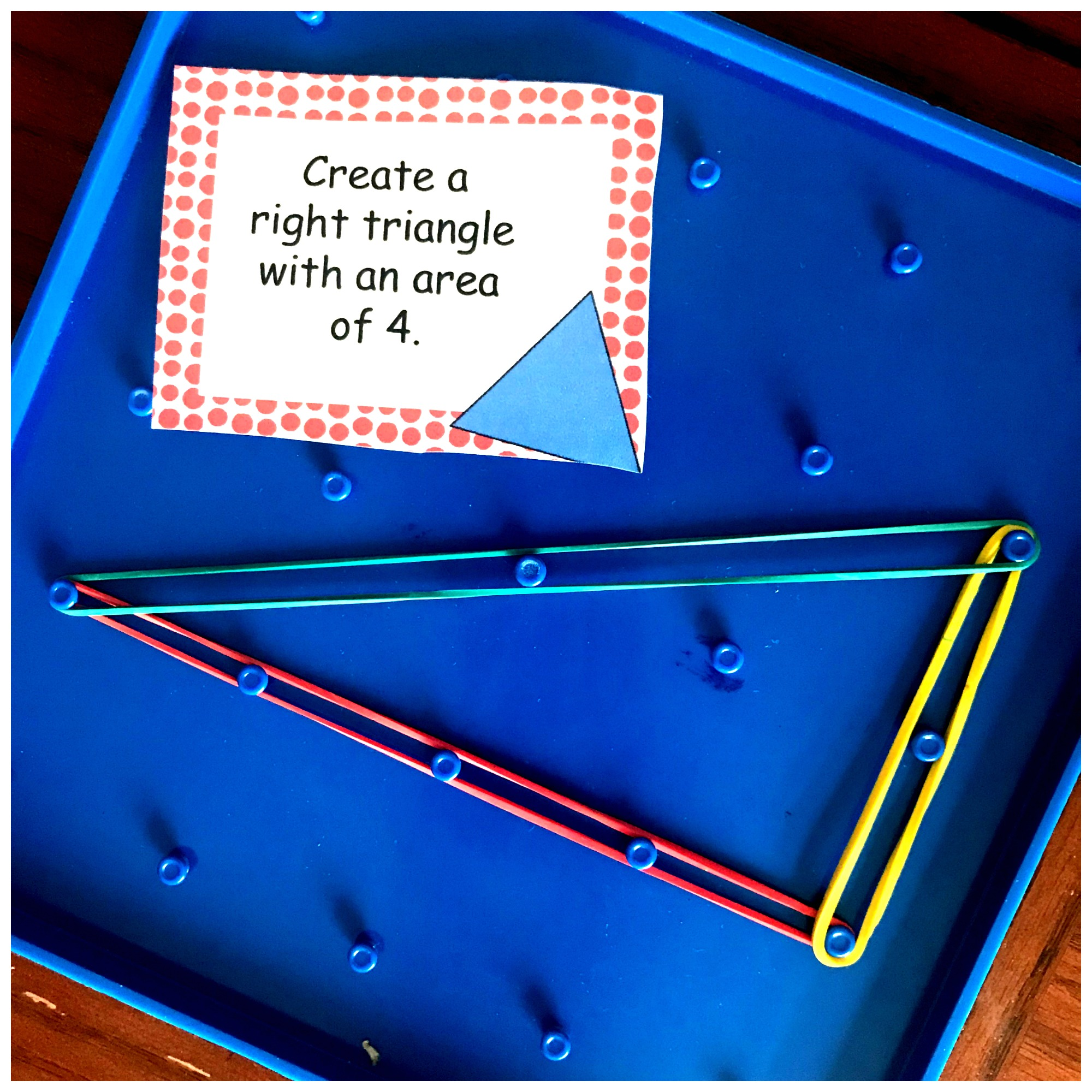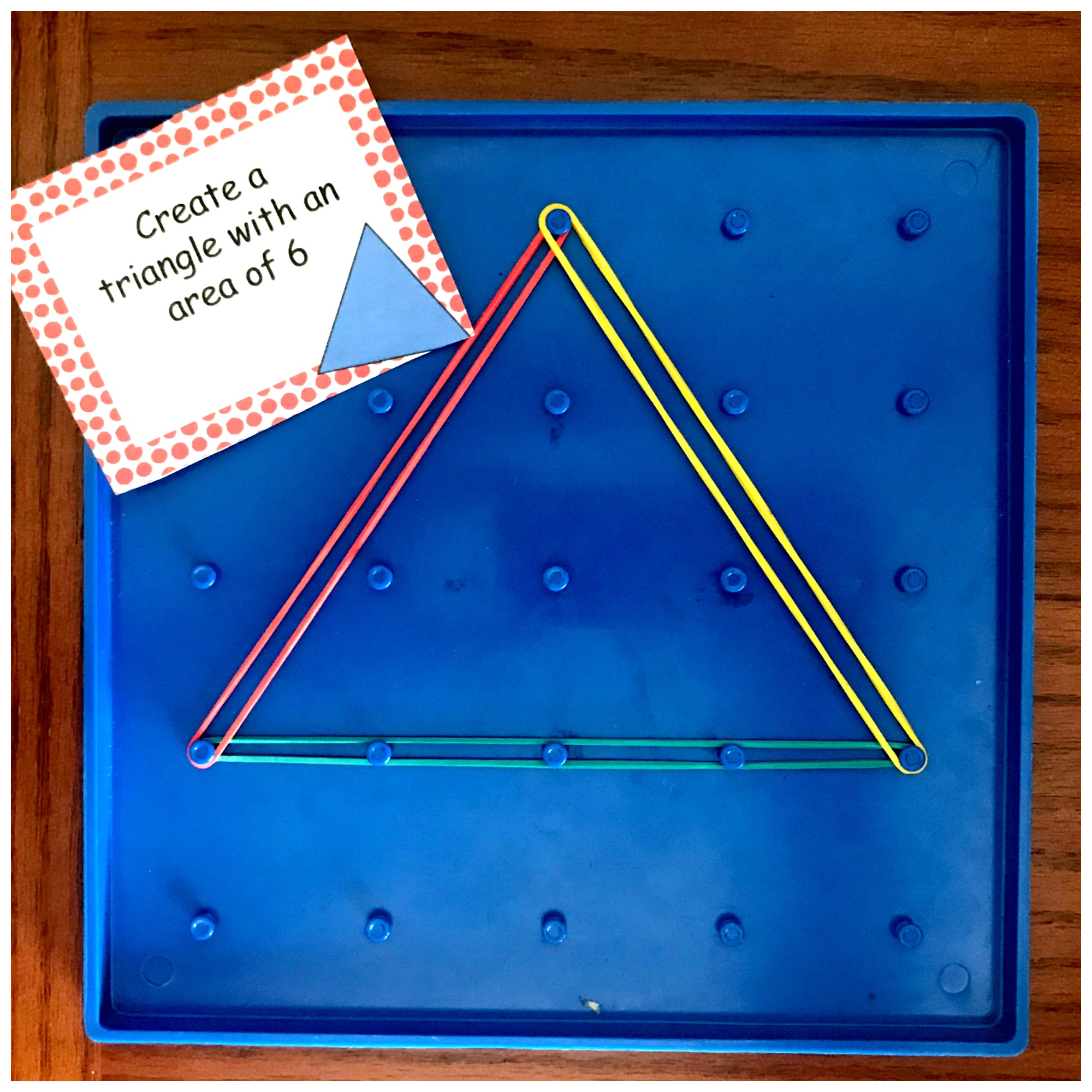Home » Math » 12 STEM Challenge Cards For Area Of Triangles Practice

# 12 STEM Challenge Cards For Area Of Triangles Practice

Need some Area of Triangles Practice? These fun STEM cards are a great way for children to practice finding the area of triangles as well as creating triangles with a given area.We are on a conquest to conquer all things area.

Our math memory work has been focusing on memorizing the area of rectangles, squares, triangles, and circles. So today, it is time for a little STEM as we work on creating triangles with a given area!

## Prep – Work

This activity requires very little prep.

1. Print off the STEM cards on card stock.
2. Cut out and laminate if desired.
3. Provide kids with geo boards and rubber bands — you could use grid paper if that is all you have. It will take a little more time, but doable.

## Area Of Triangles Practice

The concept is simple, but carrying it out will require that your kiddos understand the area of a triangle.

For the first set of cards, the children are given the area of a triangle, and they are asked to create a triangle that has that area.

#### Right Triangle:

In this example, the children know that the area should be 4. So there are two ways to find the answer.

The first way is to create a rectangle that has an area of 8, and then cut it in half. We can do this because we know from doing the interactive notebook, that a triangle has an area that is 1/2 a rectangle that has the same base and height.Or kiddos could just play with the rubber bands until they have created a triangle that has four squares in it. The trick here is for them understand that when the rubber band cuts a square in half, that they need two of those to equal one square. Yes, we are building fraction sense too!!!#### Other Triangles:

These triangles require a different kind of skill. With these, the students must understand that the height is not the side. The height MUST be a straight line from the base. The children will need to start with the answer and find factors for that number.In this example, we want to create a triangle with the area of 6.

The first step is to multiply that answer by 2…if we don’t when we use the 1/2 part of the formula we will get 3 instead of 6.

Now we have 12, and the next step is to find the factors of 12. The children can create a triangle using 12 and 1, 2 and 6, or 3 and 4.  As you can see, I created a triangle with a base of four and a height of three.

If your child needs help figuring out 1/2 of a number, this game might help.

#### Find the area of a triangle practice.

The last set of cards give children the base and height. They must build the triangle and then figure out the area.

In this example, the height is 2 and the base is 3.Once it built, we can now plug in our numbers to the formula to figure out the area.

1/2 b x h

1/2 x 2 x 3

1/2 x 6

3

As my oldest worked on building and creating his triangles, I began to see an understanding develop! I hope you see the same!

You’ve Got This

Rachel